NEET  >  Test: Coulomb's Law & Its Applications

# Test: Coulomb's Law & Its Applications

Test Description

## 10 Questions MCQ Test Physics Class 12 | Test: Coulomb's Law & Its Applications

Test: Coulomb's Law & Its Applications for NEET 2023 is part of Physics Class 12 preparation. The Test: Coulomb's Law & Its Applications questions and answers have been prepared according to the NEET exam syllabus.The Test: Coulomb's Law & Its Applications MCQs are made for NEET 2023 Exam. Find important definitions, questions, notes, meanings, examples, exercises, MCQs and online tests for Test: Coulomb's Law & Its Applications below.
Solutions of Test: Coulomb's Law & Its Applications questions in English are available as part of our Physics Class 12 for NEET & Test: Coulomb's Law & Its Applications solutions in Hindi for Physics Class 12 course. Download more important topics, notes, lectures and mock test series for NEET Exam by signing up for free. Attempt Test: Coulomb's Law & Its Applications | 10 questions in 10 minutes | Mock test for NEET preparation | Free important questions MCQ to study Physics Class 12 for NEET Exam | Download free PDF with solutions
 1 Crore+ students have signed up on EduRev. Have you?
Test: Coulomb's Law & Its Applications - Question 1

### Two insulated charged copper spheres A and B have their centers separated by a distance of 50 cm. What is the mutual force of electrostatic repulsion if the charge on each is 6.5×10−7 C? The radii of A and B are negligible compared to the distance of separation.

Detailed Solution for Test: Coulomb's Law & Its Applications - Question 1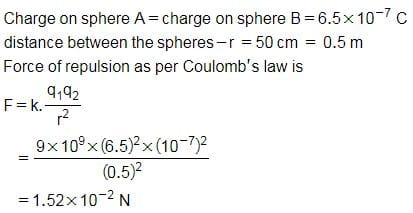Test: Coulomb's Law & Its Applications - Question 2

### Two charges 1C and -4C exists in air. What is the direction of force?

Detailed Solution for Test: Coulomb's Law & Its Applications - Question 2

Since the charges are unlike, the force will be attractive. Thus the force directs from 1C to -4C.

Test: Coulomb's Law & Its Applications - Question 3

### Ratio of the permittivity of medium to the permittivity of free space is known as

Detailed Solution for Test: Coulomb's Law & Its Applications - Question 3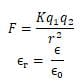Where ∈r is called the dielectric constant or relative permittivity of the medium

Test: Coulomb's Law & Its Applications - Question 4

An infinite number of charges, each equal to 4C, are placed along the x-axis at x = 1 m, x = 2 m, x = 4 m, x = 8 m and so on. The total force on a charge of 1C placed at the origin is

Detailed Solution for Test: Coulomb's Law & Its Applications - Question 4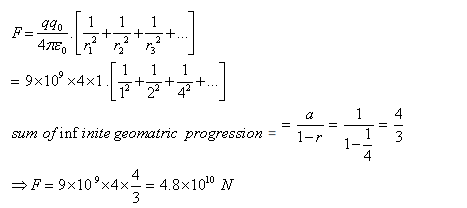Test: Coulomb's Law & Its Applications - Question 5

Two point charges 3μC and + 7μC repel each other with a force of 12 N. If each is given an additional charge -5 μC, what will be the new force between them?

Detailed Solution for Test: Coulomb's Law & Its Applications - Question 5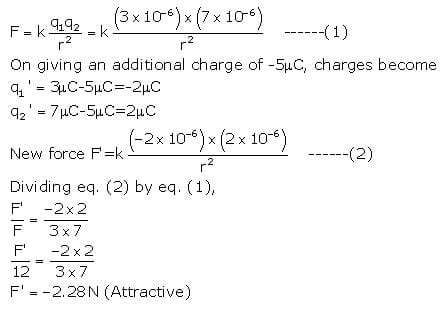Test: Coulomb's Law & Its Applications - Question 6

Electrostatic force is

Detailed Solution for Test: Coulomb's Law & Its Applications - Question 6

Electrostatics means charges at rest, or relatively at rest so this force can be applicable only when charge produce electric field which is constant which is possible only when charges at rest or relatively at rest.

Test: Coulomb's Law & Its Applications - Question 7

Two positive charges

Detailed Solution for Test: Coulomb's Law & Its Applications - Question 7

According to coulomb's law unlike charges always attract each other while like charges always repel each other so between two positive charges repulsion is working.

Test: Coulomb's Law & Its Applications - Question 8

Under the influence of the coulomb field of charge +Q, a charge −q is moving around it in an elliptical orbit. Find out the correct statement(s).

Detailed Solution for Test: Coulomb's Law & Its Applications - Question 8

Since the charge –q is moving in elliptical orbit so to make its motion stable the total angular momentum of the charge is constant since it experience a centripetal force from the charge +Q so it follow the motion as the motion of earth around sun.

Test: Coulomb's Law & Its Applications - Question 9

The force between two charges is 120 N. If the distance between the charges is doubled, the force will be

Detailed Solution for Test: Coulomb's Law & Its Applications - Question 9

Coulombs law states the force between two charged particles is directly proportional to the product of charges and inversely proportional to the square of distance between them.
So if the distance is doubled then the force would decrease 4 times.
So the new force would be 120/4=30 Newton.

Test: Coulomb's Law & Its Applications - Question 10

When the distance between two charged particle is halved, the force between them becomes

Detailed Solution for Test: Coulomb's Law & Its Applications - Question 10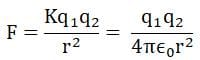## Physics Class 12

157 videos|452 docs|213 tests
 Use Code STAYHOME200 and get INR 200 additional OFF Use Coupon Code
Information about Test: Coulomb's Law & Its Applications Page
In this test you can find the Exam questions for Test: Coulomb's Law & Its Applications solved & explained in the simplest way possible. Besides giving Questions and answers for Test: Coulomb's Law & Its Applications, EduRev gives you an ample number of Online tests for practice

## Physics Class 12

157 videos|452 docs|213 tests

### How to Prepare for NEET

Read our guide to prepare for NEET which is created by Toppers & the best Teachers